# Money + interest, deposits and loan math - math problems

#### Number of problems found: 32

• Future value investmentPoseidon deposited 2,747 golden drachmas in a Mount Olympus college savings account to ensure Percy can go to college. It pays 0.04 (percent in decimal form) annual interest. After 11 years, he withdraws the money. How much more money would he have if the
• Mr. Brown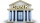Mr. Brown invested 15,000 euros in the bank for an annual term deposit. After the first year, he gained 600 euros. What was the annual interest rate of his deposit?
• If you 3If you deposit \$4500 at 5% annual interest compound quarterly, how much money will be in the account after 10 years?
• Semiannually compound interestIf you deposit \$5000 into an account paying 8.25% annual interest compounded semiannually, how long until there is \$9350 in the account?
• Compound InterestIf you deposit \$6000 in an account paying 6.5% annual interest compounded quarterly, how long until there is \$12600 in the account?
• Future valueSuppose you invested \$1000 per quarter over a 15 years period. If money earns an anual rate of 6.5% compounded quarterly, how much would be available at the end of the time period? How much is the interest earn?
• Slow saving in banksHow long will it take to save € 9,000 by depositing € 200 at the beginning of each year at 2% interest?
• Compound interest 4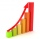3600 dollars is placed in an account with an annual interest rate of 9%. How much will be in the account after 25 years, to the nearest cent?
• Mortage hypo loan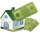The Jonáš family decided to buy an older apartment, which cost EUR 30,000. They found EUR 17,000 and took the loan with the bank for the remaining amount. What interest did they receive if they repaid this amount for 15 years at EUR 120 per month?
• Two accounts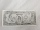Two accounts in the bank, one per year interest 2%, the second 3%. Total interest income 1900 USD. If interest rates were reversed, the yield would be USD 200 higher. What are the amounts on each account?
• Compound interest 3After 8 years, what is the total amount of a compound interest investment of \$25,000 at 3% interest, compounded quarterly? (interest is now dream - in the year 2019)
• A man 2A man divides \$10,000 into two investments, one at 10% and the other at 30%. Find how much is invested at each rate so that the two investments produce the same income annually.
• Repay, interest, loanRamchacha takes a loan amount of 240000 from a bank for constructing a house at the rate of simple interest of 12% per annum. After 1 yr. of taking the loan he rents the house at the rate of 5200 per month. Determine the number of years he would take to r
• Rate or interestAt what rate percent will Rs.2000 amount to Rs.2315.25 in 3 years at compound interest?
• Compound interestCompound interest: Clara deposited CZK 100,000 in the bank with an annual interest rate of 1.5%. Both money and interest remain deposited in the bank. How many CZK will be in the bank after 3 years?
• Interst on savings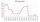The bank offers 1.6% interest. How many euros have to insert at the beginning if we received € 15 on the interest?
• The depositDeposit has been placed for one year at an annual rate of 4.5%. After the added interst amount rose to € 2,612.5. Determine initial deposit.
• Millionaire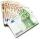Mr. Smith have gone withdraw from bank saving interest € 1,500. How big was his initial deposit if the annual interest rate is 1.5%?
• Mr. Vojta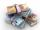Mr. Vojta put in the bank 35000 Kč. After a year bank, he credited with an interest rate of 2% of the deposit amount. How then will Mr. Vojta in the bank?
• Bank deposit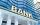Ms Jana after the withdraw amount of 2,500 euros after year saving in bank. What was her deposit if the interest rate was 2.5% p.a..

Do you have an exciting math question or word problem that you can't solve? Ask a question or post a math problem, and we can try to solve it.

We will send a solution to your e-mail address. Solved examples are also published here. Please enter the e-mail correctly and check whether you don't have a full mailbox.

Money - math problems. Interest, deposits and loan math - math problems.